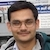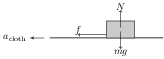# Misconceptions on Newton's Laws of MotionBy

An assertion reasoning based conceptual problem was asked in IIT JEE 2007. The problem states:

Statement 1: A cloth covers a table. Some dishes are kept on it. The cloth can be pulled out without dislodging the dishes from the table.

Statement 2: For every action there is an equal and opposite reaction.

1. Statement 1 is true, statement 2 is true; statement 2 is a correct ex- planation for statement 1.
2. Statement 1 is true, statement 2 is true; statement 2 is not a correct explanation for statement 1.
3. Statement 1 is true, statement 2 is false.
4. Statement 1 is false, statement 2 is true.

Solution: Both statements are true but statement 2 is not a correct explanation for statement 1. Generally, statement 1 is attributed to Newton's first law but this is not entirely correct. The readers are encouraged to repeat this experiment by pulling the cloth slowly. The outcome of experiment depends on acceleration $a_\text{cloth}$ of the cloth (see figure).The forces acting on the dish are its weight $mg$, normal reaction $N$, and the frictional force $f$. Maximum value of the frictional force is, \begin{align} f_\text{max}=\mu N=\mu mg. \end{align} By Newton's second law, acceleration of the dish is $a_\text{dish}=f/m$. If $a_\text{cloth}$ is small then the cloth and the dish move together i.e., $a_\text{dish}=a_\text{cloth}$. Maximum value of $a_\text{cloth}$ for the cloth and the dish to move together is given by, \begin{align} a_\text{cloth}=a_\text{dish}=f_\text{max}/m=\mu g. \end{align} Beyond this limit, $a_\text{cloth}>a_\text{dish}=\mu g$ and hence the cloth comes out leaving the dish on table.